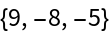#Function Repository Resource:

# DropLargestBy

Drop the largest values from a list based on a function

Contributed by: Sander Huisman  |  SHuisman
 ResourceFunction["DropLargestBy"][list,f,n] drops the n elements ei in list for which f[ei] is largest. ResourceFunction["DropLargestBy"][f,n] represents an operator form of ResourceFunction["DropLargestBy"].

## Examples

### Basic Examples (2)

Drop the largest two values:

 In:=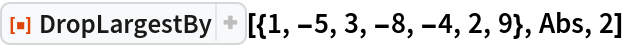Out=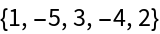Use the operator form, by first creating an operator op:

 In:=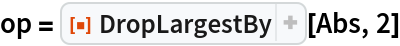Out=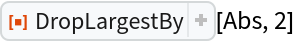Apply the operator:

 In:=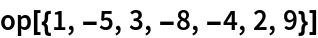Out=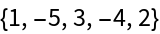### Scope (2)

With n=0 nothing is deleted:

 In:=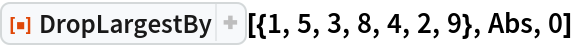Out=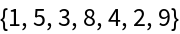A negative number deletes the smallest n numbers:

 In:=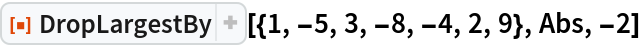Out=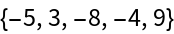### Properties and Relations (2)

If n is larger than the length of the list then an empty list is returned:

 In:=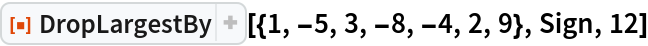Out=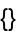DropLargestBy is complementary to TakeLargestBy:

 In:=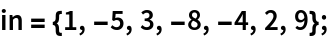In:=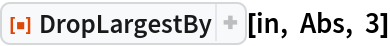Out=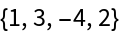In:=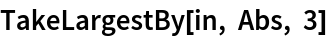Out=